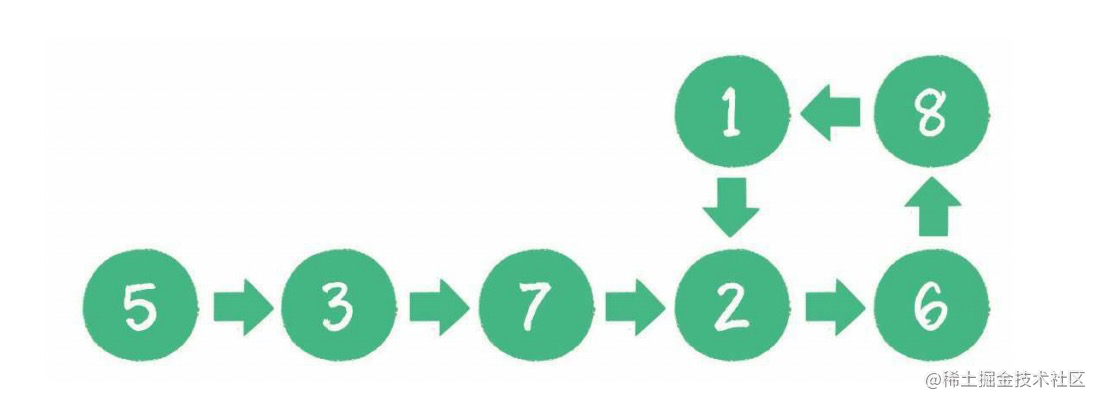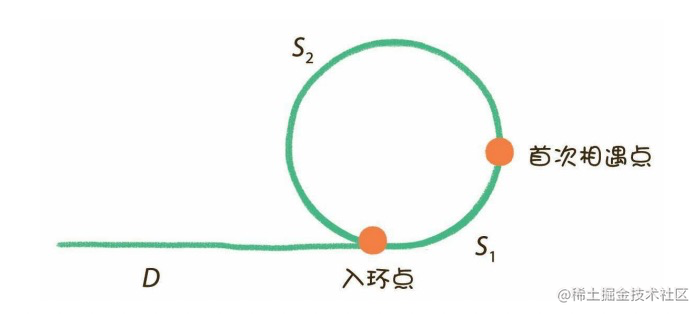## How to judge whether a linked list has links

Degree 123 2021-05-03 19:16:12
judge linked list links

If you judge that the linked list has links ？ The following listsolution 1：

Double loop traversal , Every time you traverse a new node , Just go ahead and find out if this node ever existed , Time complexity O(n2), Spatial complexity O(1)

solution 2：

But loop traversal , No traversal of a node , Just deposit the object , Traversing a new node is to find out whether the node exists in the object , Time complexity O(n), Spatial complexity O(n)

solution 3：

Set two pointers ,p1、p2, Point to the node head , Next, every time you let p1 One node forward ,p2 Two nodes ahead , If the list has rings , be p2、p1 Then it will overlap , Point to the same node

Time complexity O(n), Spatial complexity O(1)

The code is as follows ：

`````` /**
* @param {*} head Chain header node
* @description Determine if the list has links
*/
function isCycle(head:LinkNodeType | null):boolean {
let p1 = head;
let p2 = head;
while(p2!==null && p2.next !== null && p1 !== null) {
p2 = p2.next.next;
p1 = p1.next;
if(p2 === p1) {
return true
}
}
return false
};
function main() {
let node1 = new LinkNode(5)
let node2 = new LinkNode(3)
let node3 = new LinkNode(7)
let node4 = new LinkNode(2)
let node5 = new LinkNode(6)
let node6 = new LinkNode(8)
let node7 = new LinkNode(1)
node1.next = node2;
node2.next = node3;
node3.next = node4;
node4.next = node5;
node5.next = node6;
node6.next = node7;
node7.next = node5;
console.log(isCycle(node1))
}
main()
Copy code ``````

Expand ：

1. If the list has links , Find the length of the ring

solution ： When the two hands meet for the first time , Prove that a linked list has rings , Now let the pointer go on , Until the second meeting , here , The fast pointer goes one more turn than the slow one

The code is as follows ：

`````` /**
* @param {(LinkNodeType | null)} head
* @returns {(boolean|number)}
* @description Determine if the list has links , How to have a ring Find the ring length
*/
function cycleLength(head: LinkNodeType | null):boolean|number {
let p1 = head;
let p2 = head;
let isFirst = true;
let length = 0
while(p1 !== null&& p2 !== null && p2.next !== null) {
p2 = p2.next.next;
p1 = p1.next;
if(!isFirst) {
length ++
}
if(p2 == p1 && !isFirst) {
return length
}
if(p2 == p1) {
isFirst = false
}
}
return false
}
Copy code ``````

2. If the list has links , Find the access point

Explain ：

As followsp1 The distance of the pointer is D + S1

p1 The distance of the pointer is D + S1 + S2 + S1 = D + S2 + 2S1

Again because p2 The speed of the pointer is 2,p1 The speed of the pointer is 1 therefore

D + S2 + 2S1 = 2(D + S1)

D + S2 + 2S1 = 2D + 2S1

D + S2 = 2D

D = S2

therefore , When two nodes meet for the first time , Get the node , Set two pointers p1 p2 Put them in the head of the chain , And meeting nodes , Go in turn 1, Then the meeting point is Entry point

The code is as follows ：

`````` /**
* @param {*} head Chain header node
* @description Determine if the list has links
*/
function isCycle(head:LinkNodeType | null):boolean | LinkNode {
let p1 = head;
let p2 = head;
while(p2!==null && p2.next !== null && p1 !== null) {
p2 = p2.next.next;
p1 = p1.next;
if(p2 === p1) {
return p1 as LinkNode
}
}
return false
};
/**
* @param {*} firstDot The first meeting point
* @returns Entry point
*/
function cycleDot(firstDot:LinkNode,headDot:LinkNode):LinkNode {
let p1 = firstDot;
let p2 = headDot;
while(p1 !== p2) {
p1 = p1.next as LinkNode;
p2 = p2.next as LinkNode;
}
return p1
}
function main() {
let node1 = new LinkNode(5)
let node2 = new LinkNode(3)
let node3 = new LinkNode(7)
let node4 = new LinkNode(2)
let node5 = new LinkNode(6)
let node6 = new LinkNode(8)
let node7 = new LinkNode(1)
node1.next = node2;
node2.next = node3;
node3.next = node4;
node4.next = node5;
node5.next = node6;
node6.next = node7;
node7.next = node4;
let firstNode = isCycle(node1)
if(firstNode){
console.log(cycleDot(firstNode as LinkNode, node1))
}
}
Copy code ``````

Summary from : Comics algorithm Xiaohui's algorithm tour

https://qdmana.com/2021/05/20210503191542338n.html